You are currently offline. Some features of the site may not work correctly.

# Rational difference equation

A rational difference equation is a nonlinear difference equation of the form where the initial conditions are such that the denominator never… Expand
Wikipedia

## Papers overview

Semantic Scholar uses AI to extract papers important to this topic.
2016
2016
• 2016
• Corpus ID: 119133284
This paper deals with the solutions, stability character, and asymptotic behavior of the difference equation where… Expand
•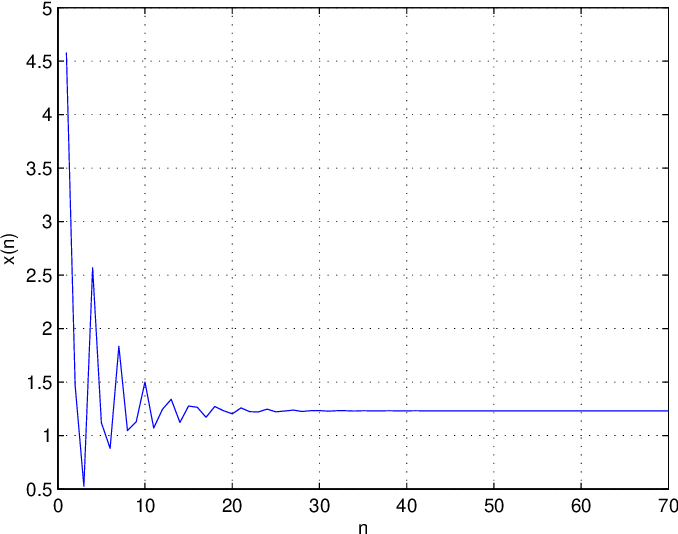•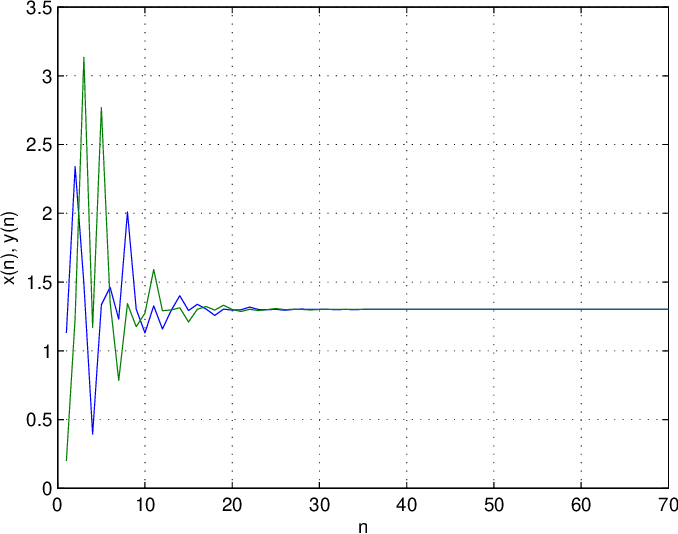Highly Cited
2009
Highly Cited
2009
• Appl. Math. Comput.
• 2009
• Corpus ID: 2860534
We describe all the solutions of a rational difference equation from Putnam's mathematical competition, which are eventually… Expand
2009
2009
• Comput. Phys. Commun.
• 2009
• Corpus ID: 29076212
In this paper, we consider the (k+1)-order rational difference equation yn+1=p+qyn+ryn−k1+yn−k,n=0,1,2,… where k∈{1,2,3,…}, and… Expand
2009
2009
Motivated by ideas from papers: C. Cinar, I. Yalcinkaya, S. Stevic, A note on global asymptotic stability of a family of rational… Expand
Highly Cited
2007
Highly Cited
2007
• S. Stevic
• Appl. Math. Lett.
• 2007
• Corpus ID: 5416037
Abstract We prove that the Putnam difference equation x n + 1 = x n + x n − 1 + x n − 2 x n − 3 x n x n − 1 + x n − 2 + x n − 3… Expand
2007
2007
• 2007
• Corpus ID: 7794991
Abstract This paper studies global asymptotic stability for positive solutions to the equation y n = y n − k y n − l y n − m + y… Expand
•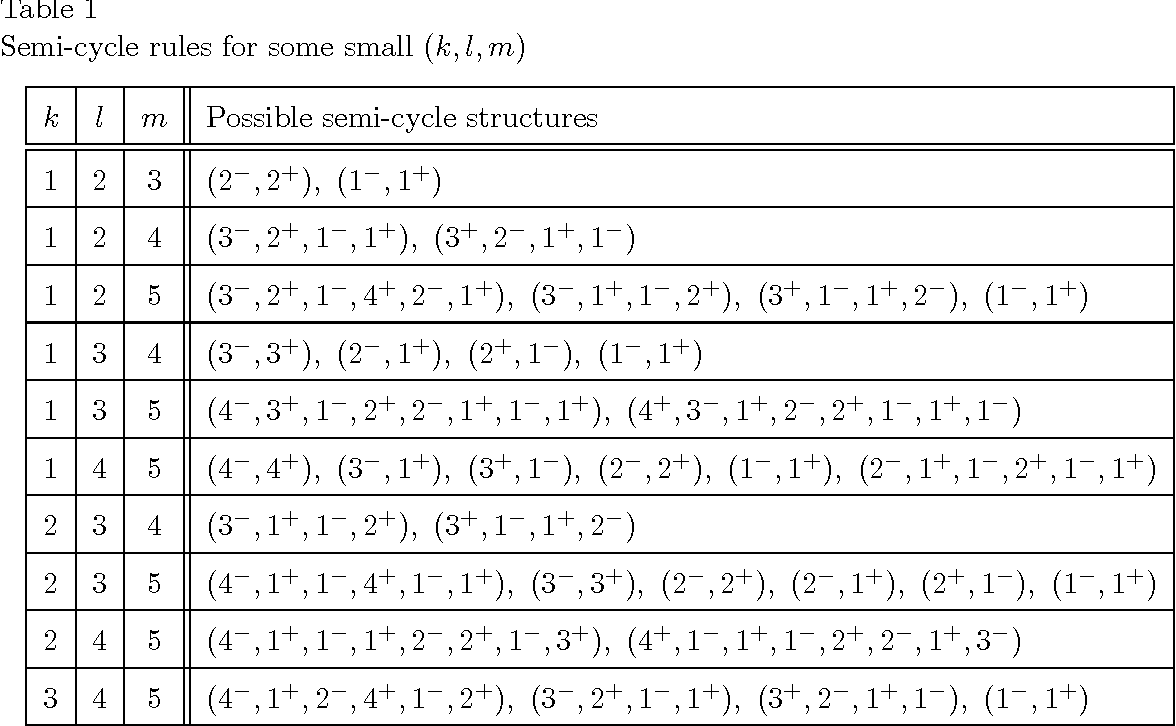2006
2006
• 2006
• Corpus ID: 42075080
We investigate in this paper the global behavior of the following difference equation: , n = 0,1,..., under appropriate… Expand
Highly Cited
2006
Highly Cited
2006
Abstract In this note, we investigate the solution of the difference equation x n + 1 = x n - 1 a - x n - 1 x n , n = 0 , 1 , 2… Expand
2006
2006
• Appl. Math. Comput.
• 2006
• Corpus ID: 41306728
Abstract In this paper, we will investigate a nonlinear rational difference equation of higher order. Our concentration is on… Expand
•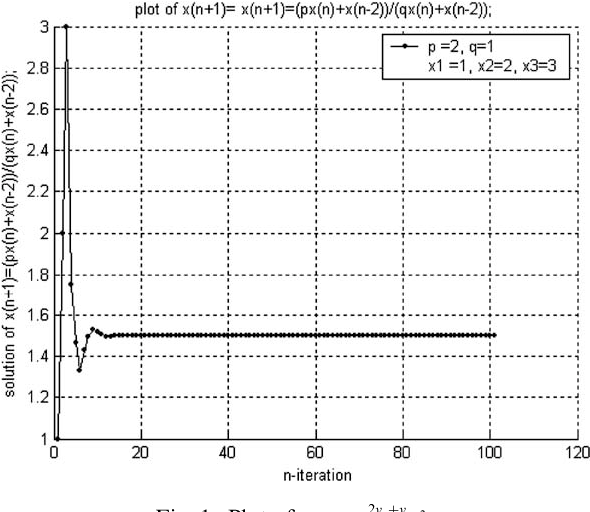•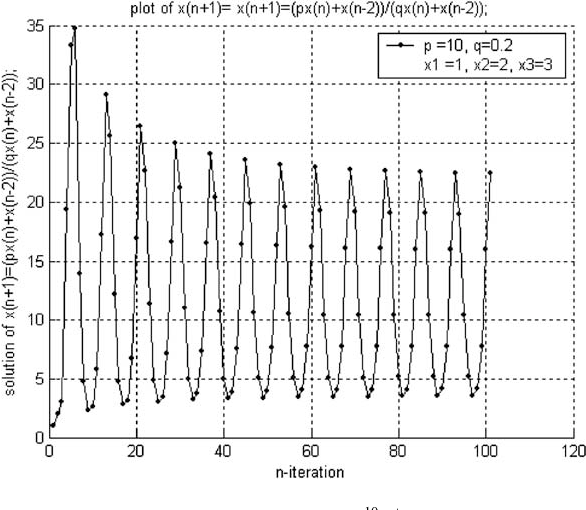•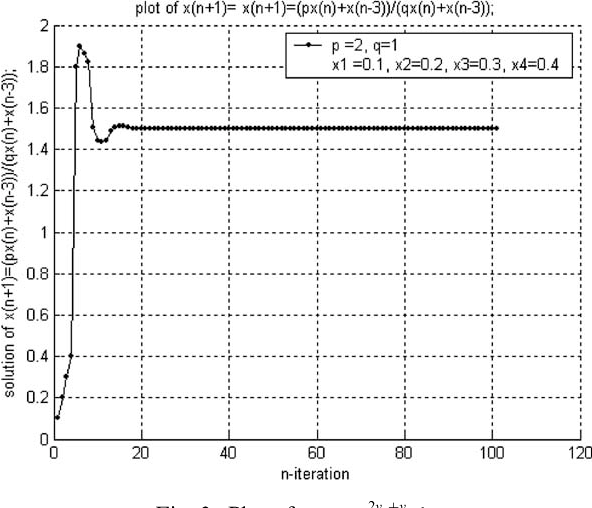•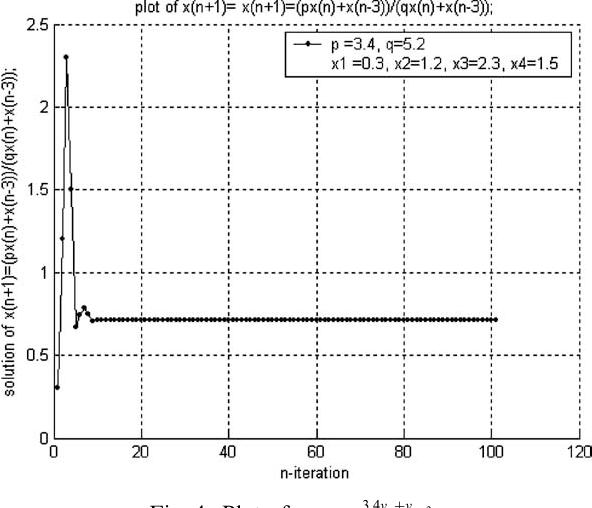2005
2005
• Appl. Math. Comput.
• 2005
• Corpus ID: 13225111
In this paper, we investigate the periodic character, invariant intervals, oscillation and global stability of all positive… Expand